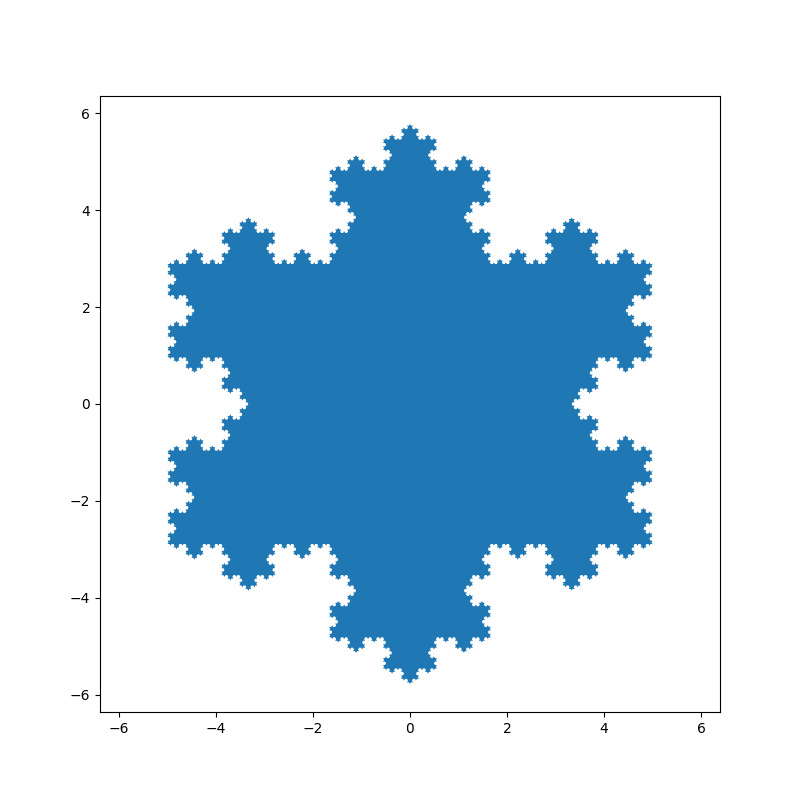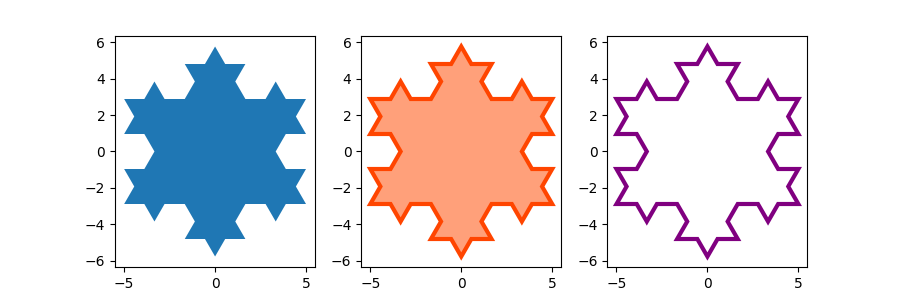# Filled polygon#

fill() draws a filled polygon based on lists of point coordinates x, y.

This example uses the Koch snowflake as an example polygon.

import numpy as np
import matplotlib.pyplot as plt

def koch_snowflake(order, scale=10):
"""
Return two lists x, y of point coordinates of the Koch snowflake.

Parameters
----------
order : int
The recursion depth.
scale : float
The extent of the snowflake (edge length of the base triangle).
"""
def _koch_snowflake_complex(order):
if order == 0:
# initial triangle
angles = np.array([0, 120, 240]) + 90
return scale / np.sqrt(3) * np.exp(np.deg2rad(angles) * 1j)
else:
ZR = 0.5 - 0.5j * np.sqrt(3) / 3

p1 = _koch_snowflake_complex(order - 1)  # start points
p2 = np.roll(p1, shift=-1)  # end points
dp = p2 - p1  # connection vectors

new_points = np.empty(len(p1) * 4, dtype=np.complex128)
new_points[::4] = p1
new_points[1::4] = p1 + dp / 3
new_points[2::4] = p1 + dp * ZR
new_points[3::4] = p1 + dp / 3 * 2
return new_points

points = _koch_snowflake_complex(order)
x, y = points.real, points.imag
return x, y


Basic usage:

x, y = koch_snowflake(order=5)

plt.figure(figsize=(8, 8))
plt.axis('equal')
plt.fill(x, y)
plt.show()Use keyword arguments facecolor and edgecolor to modify the colors of the polygon. Since the linewidth of the edge is 0 in the default Matplotlib style, we have to set it as well for the edge to become visible.

x, y = koch_snowflake(order=2)

fig, (ax1, ax2, ax3) = plt.subplots(1, 3, figsize=(9, 3),
subplot_kw={'aspect': 'equal'})
ax1.fill(x, y)
ax2.fill(x, y, facecolor='lightsalmon', edgecolor='orangered', linewidth=3)
ax3.fill(x, y, facecolor='none', edgecolor='purple', linewidth=3)

plt.show()References

The use of the following functions, methods, classes and modules is shown in this example:

Total running time of the script: ( 0 minutes 1.143 seconds)

Gallery generated by Sphinx-Gallery